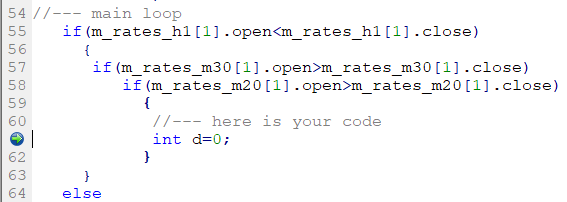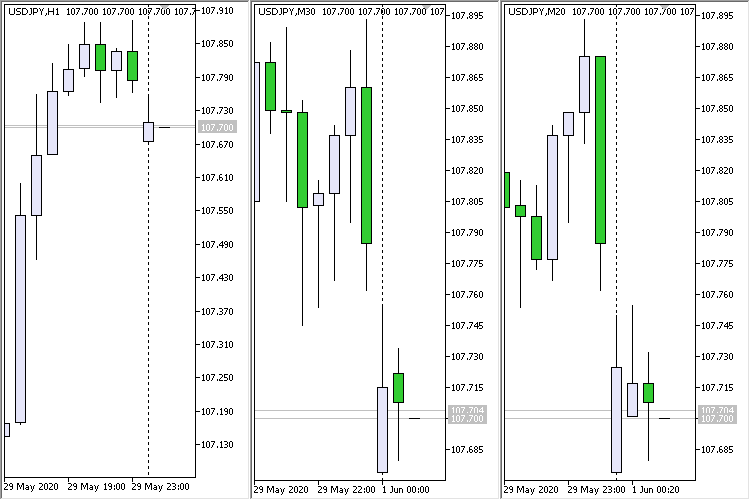# How to search for candle patterns on lower timeframes contained within a single candle in MQL5? - page 2

Jackery :
You asked me why I chose the candle on H1. I haven’t been able to figure out coding this. I manually picked it out from looking at the charts. I realised that when the upper wick is sizeable then the it is more likely that the conditions are satisfied.

How to search for candle patterns on lower timeframes contained within a single candle in MQL5?

You need to work with the CopyRates function (apply the third form - request from time to time).

```int  CopyRates(
string           symbol_name,       // symbol name
ENUM_TIMEFRAMES  timeframe,         // period
datetime         start_time,        // start date and time
datetime         stop_time,         // end date and time
MqlRates         rates_array[]      // target array to copy
);```

Use the opening time of candles on a higher timeframe as the start and end time.

Here it will be like this: 'timeframe' is the timeframe on which you will search for the pattern (for example, it will be PERIOD_M15). 'start_time' and 'stop_time' - opening time of candles from the PERIOD_H1 timeframe

as you can see, to get OHLC data you need parameters 'start_time' and 'stop_time'.

Why did you choose this particular candlestick on the H1 timeframe:

• is it (for example) index 15?
• or did it open at a specific time?
I think I misunderstood this question at first. Generally I always prefer using indexes to search for for candlestick patterns

as you can see, to get OHLC data you need parameters 'start_time' and 'stop_time'.

To be honest with you, I am having difficulty understanding how to set the CopyRates for my problem. I am definitely looking at searching the charts for the index 1 candle when it closes every hour to see if it satisfies my condition

Jackery :
To be honest with you, I am having difficulty understanding how to set the CopyRates for my problem. I am definitely looking at searching the charts for the index 1 candle when it closes every hour to see if it satisfies my condition

Clear.

We work on H1 and only at the moment of the birth of a new bar. On H1 candlestick # 1 is bullish, on M30 and on M20 candlestick # 1 is bearish. The code:

```//+------------------------------------------------------------------+
//|                                         Inside the timeframe.mq5 |
//|                                             https://www.mql5.com |
//+------------------------------------------------------------------+
#property version   "1.00"
//--- input parameters
input int      Input1=9;
//---
MqlRates m_rates_h1[],m_rates_m30[],m_rates_m20[];
datetime m_prev_bars                = 0;        // "0" -> D'1970.01.01 00:00';
//+------------------------------------------------------------------+
//| Expert initialization function                                   |
//+------------------------------------------------------------------+
int OnInit()
{
//---
ArraySetAsSeries(m_rates_h1,true);
ArraySetAsSeries(m_rates_m30,true);
ArraySetAsSeries(m_rates_m20,true);
//---
return(INIT_SUCCEEDED);
}
//+------------------------------------------------------------------+
//| Expert deinitialization function                                 |
//+------------------------------------------------------------------+
void OnDeinit(const int reason)
{
//---
}
//+------------------------------------------------------------------+
//| Expert tick function                                             |
//+------------------------------------------------------------------+
void OnTick()
{
//--- we work only at the time of the birth of new bar on H1
datetime time_0=iTime(Symbol(),PERIOD_H1,0);
if(time_0==m_prev_bars)
return;
m_prev_bars=time_0;
//--- PERIOD_H1
int start_pos=0; // start position
int count=3; // data count to copy
if(CopyRates(Symbol(),PERIOD_H1,start_pos,count,m_rates_h1)!=count)
return;
//--- PERIOD_M30
if(CopyRates(Symbol(),PERIOD_M30,m_rates_h1.time,m_rates_h1.time,m_rates_m30)!=3)
return;
//--- PERIOD_M20
if(CopyRates(Symbol(),PERIOD_M20,m_rates_h1.time,m_rates_h1.time,m_rates_m20)!=4)
return;
//--- main loop
if(m_rates_h1.open<m_rates_h1.close)
{
if(m_rates_m30.open>m_rates_m30.close)
if(m_rates_m20.open>m_rates_m20.close)
{

}
}
else
{
return;
}
}
//+------------------------------------------------------------------+
```

put a breakpoint here:and the result is:Clear.

We work on H1 and only at the moment of the birth of a new bar. On H1 candlestick # 1 is bullish, on M30 and on M20 candlestick # 1 is bearish. The code:

put a breakpoint here:

and the result is:

Thanks so much. This is precisely what I need. I now have a better understanding of CopyRates(). 🙏